# Math in Focus Grade 2 Chapter 13 Answer Key Customary Measurement of Length

This handy Math in Focus Grade 2 Workbook Answer Key Chapter 13 Customary Measurement of Length detailed solutions for the textbook questions.

## Math in Focus Grade 2 Chapter 13 Answer Key Customary Measurement of Length

Challenging Practice

Question 1.
There are three drawings, A, B, and C. Drawing A is shown.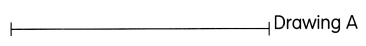Drawing B is 12 inches longer than Drawing A. Drawing C is 4- inches shorter than Drawing B. How long is Drawing C?

Given,

Drawing B is 12 inches longer than Drawing A.

Drawing C is 4- inches shorter than Drawing B.

Length of Drawing C = 12 + 4 = 16

Therefore, Drawing C is 16 inches long.

Question 2.
Tom and Lionel climb a tree. They have to climb a ladder first, then up to the branches. The ladder is 6 feet long. Tom climbs 4- feet from the ladder to a branch. Lionel climbs 2 feet from the ladder to another branch. What is the total distance that Tom and Lionel have climbed?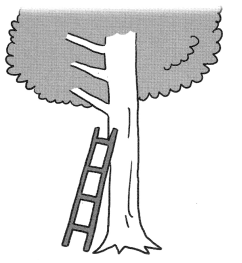Given,

Tom and Lionel climb a tree.

They have to climb a ladder first, then up to the branches.

The ladder is 6 feet long.

Tom climbs 4- feet from the ladder to a branch.

Lionel climbs 2 feet from the ladder to another branch.

Distance climbed by Tom = 6 + 4 = 10 feet

Distance climbed by Lionel = 6 + 2 = 8 feet

Total distance = 10 + 8 = 18 feet

Therefore, the Total distance climbed by Tom and Lionel is 18 feet.

Problem Solving

Solve.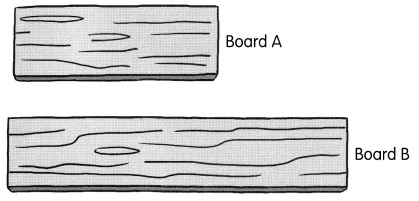Question 1.
There are 2 boards, A and B. The total length of both boards is 28 feet. Board B is at least 5 feet longer than Board A, but the difference is not more than 12 feet. What are the possible lengths of the two boards?

Given,

There are 2 boards, A and B.

The total length of both boards is 28 feet.

Board B is at least 5 feet longer than Board A, but the difference is not more than 12 feet.

Let Length of Board  A be a

Length of Board B be b

From thee given data, these conditions can be drawn,

a + b = 28

b  ≥ a + 5

b – a < 12

So,  substituting a = 28 – b in b  ≥ a + 5, it becomes,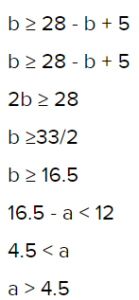Therefore,

The possible lengths of board a is 8 to 11.5 feet.

The possible lengths of board b is 16.5 to 20 feet.

Chapter Review/Test

Vocabulary

Fill in the blanks with words from the box.

foot/feet
inch/inches
tallest
shorter

Question 1.
Measure short lengths in ___________ and longer lengths in __________

Measure short lengths in foot/feet and longer lengths in inch/inches.

Question 2.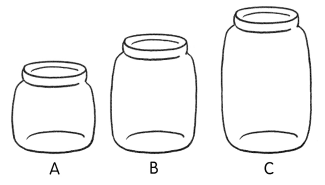Cookie jar C is the __________

Cookie jar C is the tallest.

Question 3.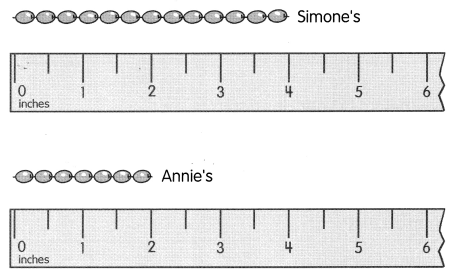Annie’s string of beads is 2 inches __________ than Simone’s.

Annie’s string of beads is 2 inches shorter than Simone’s.

Concepts and Skills

Question 4.
What is the length of your math textbook?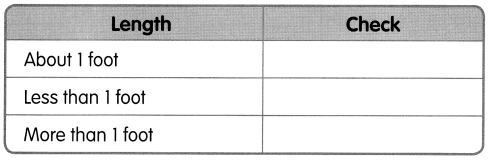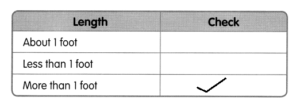Question 5.
What is the height of your desk?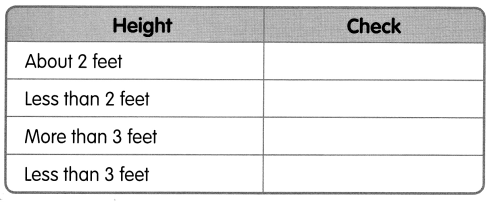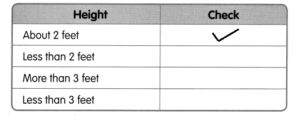Question 6.
What is the height of your classroom?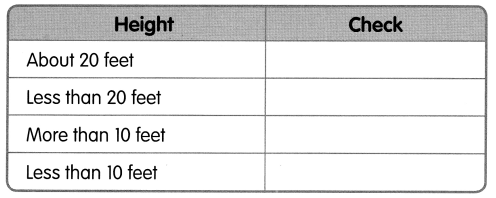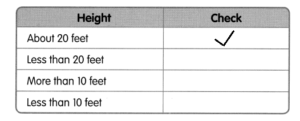Look at the objects measured. Then fill in the blanks.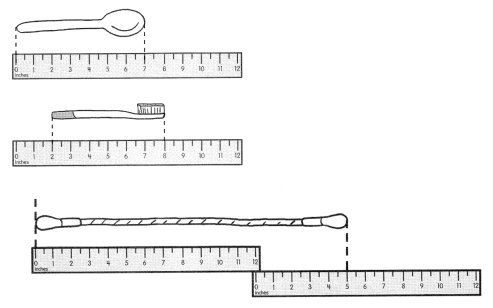Question 7.
spoon: __________ in.

Spoon : 7 in

Question 8.
toothbrush: __________ in.

toothbrush : 8 – 2 = 6 in

Question 9.
rope: __________ ft __________ in.

rope : 1 ft 5 in

Question 10.
The rope is __________ foot longer than the spoon.

The rope is 0.8 foot longer than the spoon.

Question 11.
The rope is longer than __________ foot.

The rope is longer than 1 foot.

Question 12.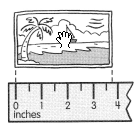The photo is about __________ inches long.

The photo is about 4 inches long.

Question 13.
Use a ruler to draw a part of a line 7 inches long.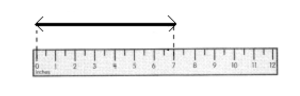Problem Solving

Question 14.
Ms. Cooper used 86 feet of yellow yarn and 123 feet of blue yarn to make a sweater.
a. What is the total length of yarn that Ms. Cooper used to make a sweater?
The total length of yarn is __________ feet.

Given,

Ms. Cooper used 86 feet of yellow yarn and 123 feet of blue yarn to make a sweater.

Total length = 86 + 123 = 209 feet

Therefore, The total length of yarn is 209 feet.

b. How much more blue yarn than yellow yarn did Ms. Cooper use?
Ms. Cooper used ____________ feet more blue yarn than yellow yarn.

Given,

Ms. Cooper used 86 feet of yellow yarn and 123 feet of blue yarn to make a sweater.

Difference = 123 – 86 = 37 feet

Therefore, Ms. Cooper used 37 feet more blue yarn than yellow yarn.

Question 15.
A bookshelf is 50 inches tall. It is 15 inches shorter than a step ladder. How tall is the ladder?
The step ladder is ____________ inches tall.